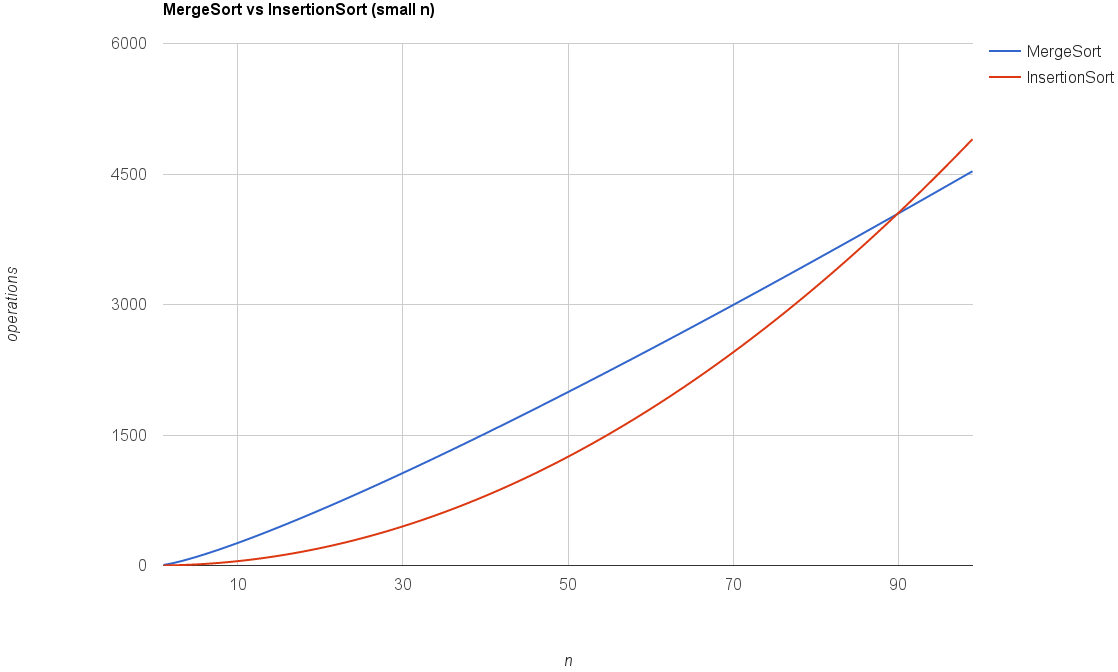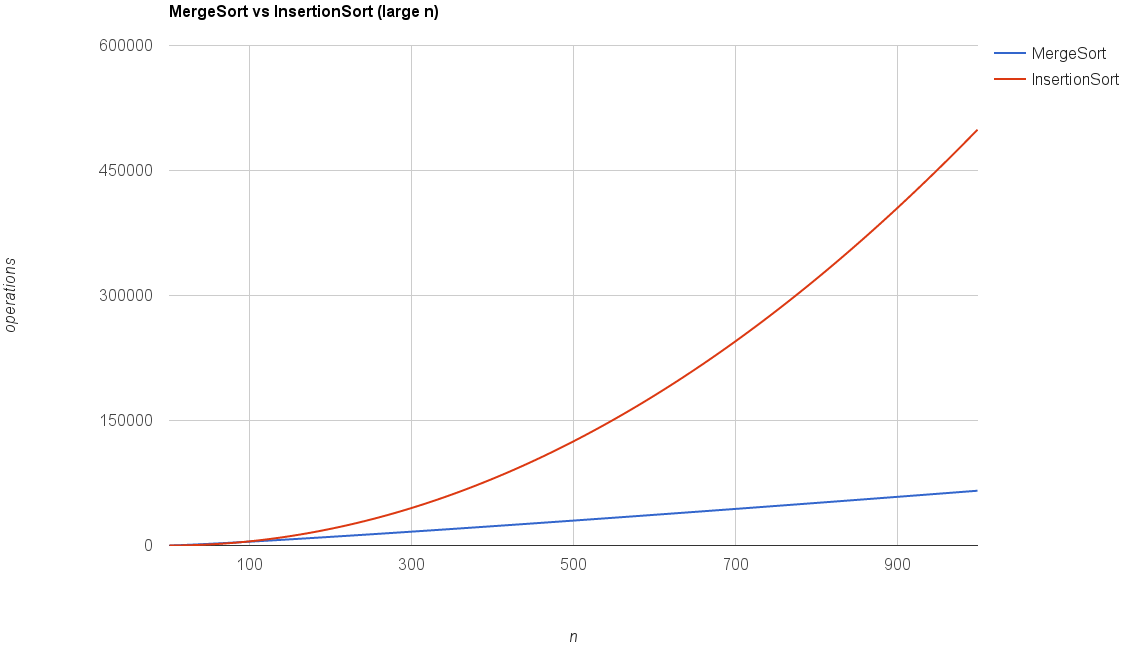## monkey codes

Random bits of knowledge, functional code examples and laughable mistakes from a code monkey working in the real world.

Curious software developer, motorcycle enthusiast, rugby fanatic and biltong connoisseur. My code always works sometimes.

# Analyzing The Merge Sort Algorithm

An example of how to analyze the running time of a divide and conquer algorithm like merge sort.

## Strategy - Divide and Conquer

Taking a problem, breaking it down into smaller problems that you solve recursively and then combine the results of the smaller sub problems to get a solution to the original problem.

## Example

### pseudo code

C = Output Array [length = n]
A = Left Sorted Array [length = n/2]
B = Right Sorted Array [length = n/2]
i = 0 [Pointer in A] # 1 op
j = 0 [Pointer in B] # 1 op

for K = 1 to n       # 1 op
if A[i] < B[j]      # 1 op
C[K] = A[i]       # 1 op
i++               # 1 op OR same amount in else
else B[j] < A[i]
C[K] = B[j]
j++
end


### Analysis

To calculate the total number of operations ("ops" in pseudo code), first calculate the operations at each level of recursion, excluding the recursion to deeper levels. This only leaves the merge step at each level. Counting the number of operations for the merge in the pseudo code breaks down to $4m + 2$ ( 4 ops in the loop and 2 to setup i and j ) for an array of $m$ numbers, which can be simplified to $6m$ since $m \geqslant 1$.

The recursive nature of the algorithm can be represented as a binary tree, each node shows the level (L0, L1, L2...) followed by the size of the input at that level (8, 4, 2...).As the diagram depicts, given input array $n = 8$, the number of levels are $\log_2 n + 1$. At each level $j=0,1,2,...,\log_2n$ there are $2^j$ sub-problems, each with input size $\dfrac{n}{2^j}$. From the earlier calculation the merge takes $6m$ operations. To calculate for level $j$

\begin{align} m& =\dfrac{n}{2^j} \ op_j& =2^j \times 6m \ & = 2^j \times 6\left(\dfrac{n}{2^j}\right) \ & = 6n \end{align}

This means that the number of operations at any given level are independent of the level. The total can be calculated by multiplying the number of levels with the amount of work done at each level:

\begin{align} total& =6n \times (\log_2 n + 1) \ & = 6n \log_2 n + 6n \end{align}

## Guiding Principles For Analyzing Algorithms

### Principle #1 - Worst Case Analysis

The running time bound holds for every input of length $n$, especially for large $n$.

### Principle #2 - Don't Sweat The Small Stuff

Don't pay too much attention to constant factors and lower-order terms. Like the analysis of merge part was simplified from
\begin{align} \text{op_merge}& = 4m + 2 \ & = 6m\ (since\ m\ \geqslant 1) \end{align}

Using accurate constants depends on the architecture, compiler and the programming language. Algorithm analysis generally happens at a higher level. Secondly it simplifies the analysis while sacrificing very little in terms of predicting the running time of an algorithm.

### Principle #3 - Asymptotic Analysis

Focus on the running time of very large input sizes while suppressing constant factors (too system dependent) and lower order terms (irrelevant for large inputs).
$6n \log_2 n +6n$ is "better than" $\dfrac{1}{2}n^2$ (Insertion Sort) only holds for large $n$.#### "Big Oh" Notation

Provides a notation or vocabulary for expressing the analysis of an algorithm. It applies the high level idea of Asymptotic Analysis, which is to suppress constant factors and lower order terms. Applied to the merge sort analysis, $6n \log_2 n +6n$ would simply be $O(n\ log\ n)$ (Terminology: Running time is $O(n\ log\ n)$

#### "Big Oh" Examples

Does Array A contain integer T?

for i=1 to n
if A[i] == T return TRUE
return FALSE


Has a running time of $O(n)$ or linear in the input length $n$.

Does Array A or B contain integer T?

 for i=1 to n
if A[i] == T return TRUE
for i=1 to n
if B[i] == T return TRUE
return FALSE


Since there are a constant of 2 loops, independent of length $n$ it gets suppressed, $O(n)$

Does Array A and B have a number in common?

for i=1 to n
for j=1 to n
if A[i] == B[j] return TRUE
return FALSE


Has a running time of $O(n^2)$ or is said to be a quadratic time algorithm because there's a total of $n^2$ iterations.

#### What is a "Fast" Algorithm?

This can be loosely defined as an Algorithm whose worst case running time grows slowly with input size.

#### Common Functions

The most common list of functions in asymptotic analysis, listed from slowest growing to fastest growing:

1. $O(1)$
2. $O(log_2\ n)$
3. $O(n)$
4. $O(n\ log_2\ n)$
5. $O(n^2)$
6. $O(n^2\ log_2\ n)$
7. $O(n^3)$
8. $O(2^n)$

## Master Method

This can be used as a formula to calculate the running time of a recursive algorithm.

$$T(n) \leqslant aT\left(\dfrac{n}{b}\right) + O(n^d)$$

Where $a$ is the number of recursive calls or sub-problems, $b$ is the factor by which the input size shrinks on each recursive call and $d$ is the exponent in running time of the work done outside of the recursive call.

\begin{align} T(n) & = O(n^d\ log\ n) & \text{ if } a = b^d \text{ (case 1)} \ T(n) & = O(n^d) & \text{ if } a < b^d \text{ (case 2)} \ T(n) & = O(n^{log_b\ a}) & \text{ if } a > b^d \text{ (case 3)} \end{align}

In Case 1 the work remains the same at each level, Case 2 the time is dominated by the work done in the root node and in Case 3 the time is dominated by the work done in the leaf nodes.

The number of leaves of a recursion tree can be calculated as:

\begin{align} leaves & = a^{log_b\ n} \ & = n^{log_b\ a} \end{align}

### Example - Apply Master Method to Merge Sort

In merge sort the number of recursive calls ($a$) is 2 (left and right side). The input size ($b$) in each of the recursive calls is halved ($b=2$). The work done at each level ($d$) is linear (1).
\begin{align} a & = 2 \ b & = 2 \ d & = 1 \ a & = b^d ;;;;;;\text{ (case 1)} \ 2 & = 2^1 \ T(n) & = O(n^d\ log\ n) \ & = O(n\ log\ n) \end{align}

Thus the Master Method conveniently yields the same result as the original analysis.

Algorithms: Design and Analysis, Part 1